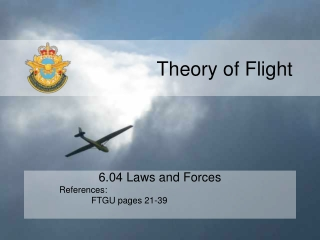DownloadDownload PresentationTheory of Flight

# Theory of Flight

Download Presentation## Theory of Flight

- - - - - - - - - - - - - - - - - - - - - - - - - - - E N D - - - - - - - - - - - - - - - - - - - - - - - - - - -
##### Presentation Transcript

1. Theory of Flight 6.04 Laws and Forces References: FTGU pages 21-39

2. Review 1. What is the secondary effect of roll and how is it created? 2. What is the secondary effect of yaw and how is it created? 3. What are the purpose of flaps? 4. What effects do spoilers have on an airplane? 5. What is trim used for?

3. 6.04 Laws and Forces • MTPs: • Newton’s Laws of Motion • Four Forces • Equilibrium • Aerodynamic Couples

4. Newton’s First Law • Newton’s First Law • An object either is at rest or maintains uniform motion, unless acted upon by an unbalanced external force

5. Newton’s Second Law • Newton’s Second Law • Force = mass x acceleration

6. Newton’s Third Law • Newton’s Third Law • For every action, there is an equal and opposite reaction. The force of the combusting rocket fuel forcing exhaust from the rocket produces an equal and opposite reaction force in the form of thrust This basic premise holds true for all propulsion

7. Newton’s First Law • What does Newton’s First Law have to do with aircraft? • Uniform motion • Straight glide/cruise • Unbalanced forces • Spoilers • Ailerons • Elevator • Rudder • Change in engine power • Flaps • Law #1 • An object either is at rest or maintains uniform motion, unless acted upon by an unbalanced external force

8. Newton’s Second Law • What does Newton’s Second Law have to do with aircraft? • Weight and balance • What is one of the main differences you will notice when you go solo? You will lift off sooner! WHY? Law #2: Force = Mass X Acceleration

9. Newton’s Third Law • What does Newton’s Third Law have to do with gliders? • Movement of control surfaces push air and cause equal and opposite reactions to allow gliders to manoeuvre • Downwashof air flowing over surface of wing causes equal and opposite reaction = LIFT • Law #3 • For every action, there is an equal and opposite reaction.

10. Four Forces • What are the four forces that act on a airplane? • Lift • Drag • Thrust • Weight

11. Equilibrium • Equilibrium: • A state in which opposing forces or influences are balanced. • Steady motion (ie. straight and level flight). • What happens when the four forces are not in equilibrium?

12. Equilibrium Is an aircraft in a state of equilibrium when it is on the ground? No - because lift does not equal weight

13. Aerodynamic Couples • Aerodynamic Couples • Parallel forces that do not pass through the same point • Couples cause a turning moment • Imagine pulling on one of the arrows, what will happen?...It will cause it to turn

14. Moment • What is a moment? • Force at a distance • Think of a teeter-totter… • Pretend you are riding a teeter-totter with a friend who is much heavier than you • Do you need to sit closer or further away to be in equilibrium?

15. Moment • Teeter-Totter Example “Force at a distance”

16. Aerodynamic Couples Aerodynamic Couples Lift and Weight • When lift > weight the aircraft will climb • When lift<weight the aircraft will descend Thrust and Drag • When drag > thrust the aircraft will slow down • When drag < thrust the aircraft will speed up

17. Aerodynamic Couples • What happens when…. • Lift is greater than thrust? • Weight is greater than drag?

18. Confirmation Check

19. Confirmation 1. What are Newton’s 3 laws? 2. What does it mean for an aircraft to be in a state of equilibrium? 3. What is the definition of a couple?

20. Confirmation • What is happen to an aircraft with the following couples?

21. Sir Isaac Newton